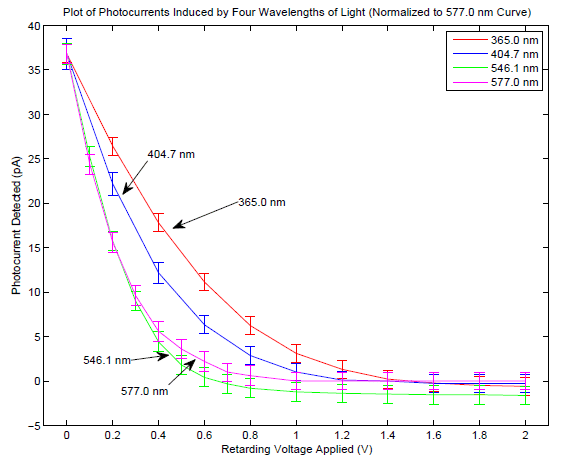# Photoelectric effect : retarding potential with back current

crick
In the experiment of the determination of ##h## using the photoelectric effect produced by light emitted by led's there is the systematic problem of the "dark current" or "back current", i.e. the current caused by photoelectric effect on the anode of the system which is used in the expreriment.

This current is opposite to the "normal" photocurrent and its effect is to make the I-V curves not going but keep decreasing (slightly).Suppose that one wants to determine $h$ using two different led's of frequencies ##\nu_1## and ##\nu_2## using the following method (I know about other methods but I would like to know about this one).

Let's call ##V_{0_1}## and ##V_{0_2}## the values of ##V## where the (total) photocurrent is ##0## (for led ##1## and led ##2##). And suppose that the relation used is $$h=\frac{e(V_{0_1}-V_{0_2})}{(\nu_2-\nu_1)} \tag{1}$$

(work function is not involved at all)

My question is: under what theoretical assymptions and explanations is ##(1)## suitable to calculate ##h## (i.e. when to determine ##V_0##, even if current does not go asymptitically to zero but becomes negative, is still a good method to determine $h$ using ##(1)##)?

My guess is that this is a "good" method only if ##V_0##Vis such that it "brakes" all the electrons always in the same way, i.e. it makes them reach the same kinetic energy, for any frequency ##\nu## that is being used.

But how is this confermed/denied theoretically?

Staff Emeritus
In the experiment of the determination of ##h## using the photoelectric effect produced by light emitted by led's there is the systematic problem of the "dark current" or "back current", i.e. the current caused by photoelectric effect on the anode of the system which is used in the expreriment.

This current is opposite to the "normal" photocurrent and its effect is to make the I-V curves not going but keep decreasing (slightly).
View attachment 200294

Suppose that one wants to determine $h$ using two different led's of frequencies ##\nu_1## and ##\nu_2## using the following method (I know about other methods but I would like to know about this one).

Let's call ##V_{0_1}## and ##V_{0_2}## the values of ##V## where the (total) photocurrent is ##0## (for led ##1## and led ##2##). And suppose that the relation used is $$h=\frac{e(V_{0_1}-V_{0_2})}{(\nu_2-\nu_1)} \tag{1}$$

(work function is not involved at all)

My question is: under what theoretical assymptions and explanations is ##(1)## suitable to calculate ##h## (i.e. when to determine ##V_0##, even if current does not go asymptitically to zero but becomes negative, is still a good method to determine $h$ using ##(1)##)?

My guess is that this is a "good" method only if ##V_0##Vis such that it "brakes" all the electrons always in the same way, i.e. it makes them reach the same kinetic energy, for any frequency ##\nu## that is being used.

But how is this confermed/denied theoretically?

Please note that for Eq. 1 to be valid, you must have a linear relationship between the stopping potential and frequency. That equation is nothing more than calculating the slope of V vs frequency.

Unfortunately, since you only have two data points, there is no way for you to verify if this relationship is valid. It is valid "on paper" per the Einstein's photoelectric effect equation, but it is not known if it is valid in your experiment due to the inadvertent photoemission off the anode. And as far as experimental methodology goes, it is a BAD experiment to only collect two data points.

I do not know to what extent the negative value of the stopping potential will affect the result. If you can show, with more data, that what you have can be represented by a linear fit, then the negative potential will not matter since all you care about is the slope of this line.

Zz.